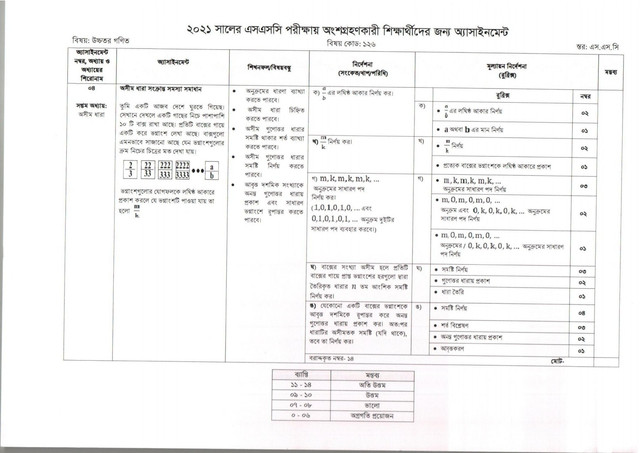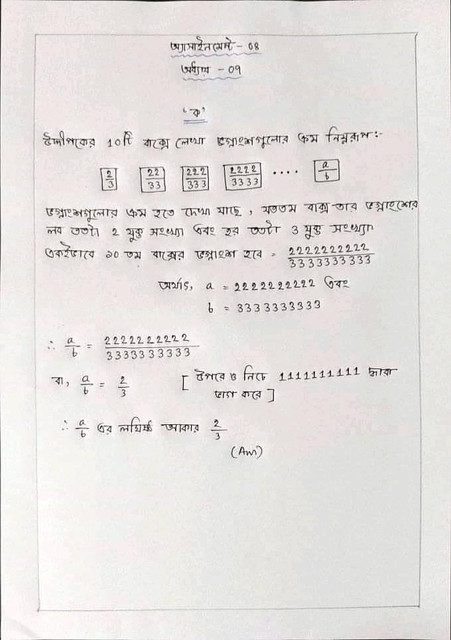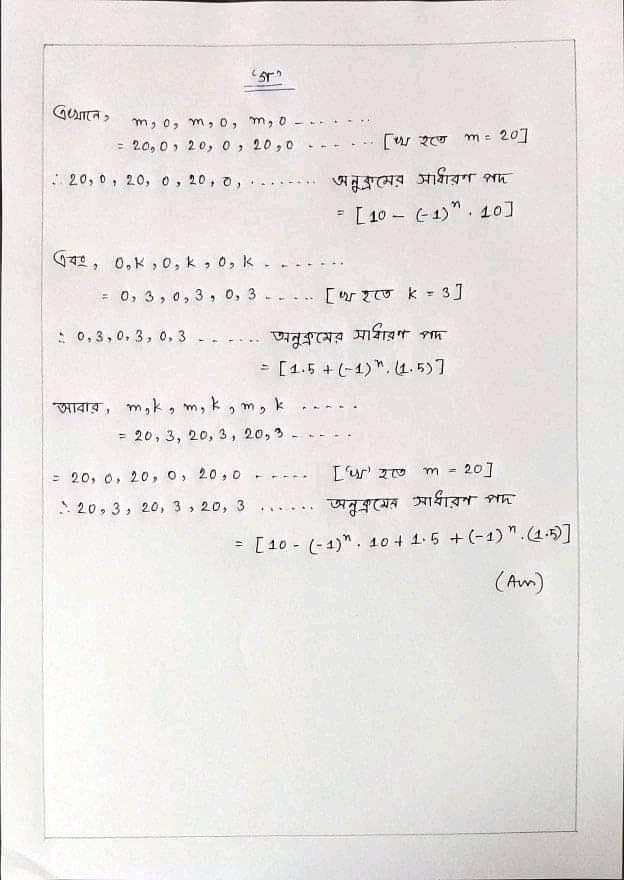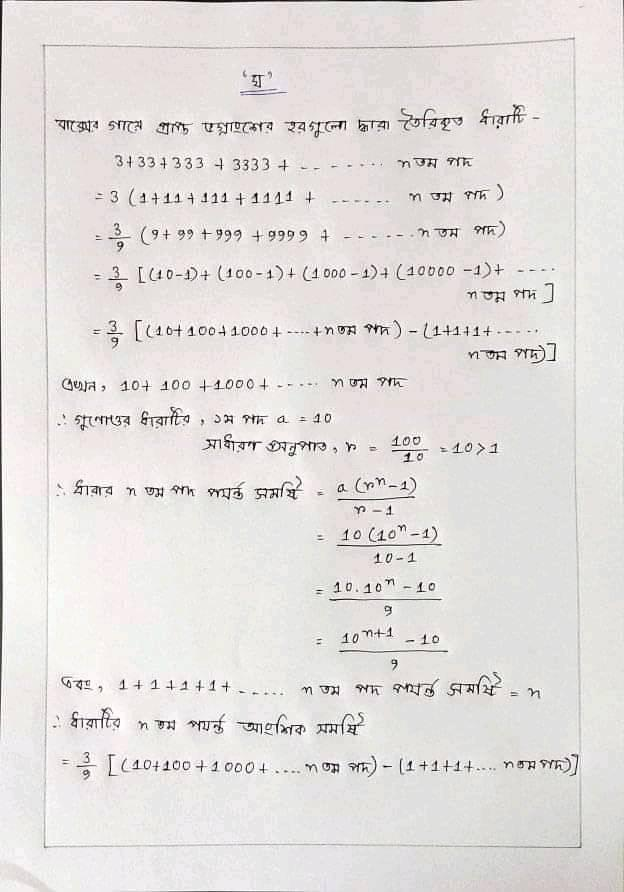Assignment

# 6th Week Higher Math Assignment Answer SSC 2023

The SSC 2023 sixth week assignment has been published in the light of the short syllabus of the 2023 SSC candidates prepared by the National Curriculum and Textbook Board as per the instructions of the Ministry of Education due to the deadly coronavirus. On 23 August 2023, the authority published the sixth week assignment of students from all public and private educational institutions of the country to participate in the SSC examination in 2023 on the website of the department.

According to the grid of assignment publication, the Department of Secondary and Higher Education published the assignment for the fifth week of SSC 2023 on 16 August 2023, following which assignment homework was given for the scheduled subjects for the sixth week on 23 August. [ বাংলায় দেখুন ]

## SSC 6th Week Assignment 2023

Assignments of the week prepared in the light of the short syllabus prescribed for the SSC candidates of 2023 have been assigned to the SSC candidates of all the Boards of Education for the sixth week from Geography and Environment, Chemistry, Accounting, Economics, Biology, Finance and Banking, Politics and Citizenship, Higher Mathematics text books.

Students will collect assignments from educational institutions in accordance with proper hygiene rules from 24th August 2023 and submit the relevant subject to the teacher by 29th August 2023 after completing the assignment writing guidelines given by the Department of Secondary and Higher Education.

Friends of 2023 Science Department SSC Examiners, Assignments for Higher Mathematics in the sixth week of the 2023 SSC Examinations for you to solve the problems related to Infinite Section; I came up with an article about SSC 2023 Higher Math Solution. Hopefully by following this you will be able to complete the higher math assignment solution of the sixth week of 2023 SSC exam very nicely.

## 6th Week Assignment Higher Math SSC 2023Assignment: Solve problem related to infinite series;

You have gone to a strange country. There are 10 boxes under a tree. Each box has a fraction written on it. The boxes are arranged in such a way that the order of the fractions looks like the figure below;

The fraction obtained by expressing the product of fractions in the smallest form is m / k

Instructions (signal / step / circumference):

A) Determine the minimum size of a / b; (Determine the value of a or b;)

B) determine m / k; (Express the fraction of each box in minimal form;)

C) Find the general terms of the sequence m, k, m, k, m, k,; (1,0,1,0,1,0,… and 0,1,0.1,0,1, করবে will use two common terms in the sequence;)

D) If the number of boxes is infinite, find the nth partial sum of the series formed by the fractions of the fraction obtained on each box;

E) Fractions of a box anywhere. Convert the recurring decimal to the infinite multiplicative series, then find the infinite sum of the series (if any), then determine it;

## SSC 2023 6th Week Higher Math Assignment Answer